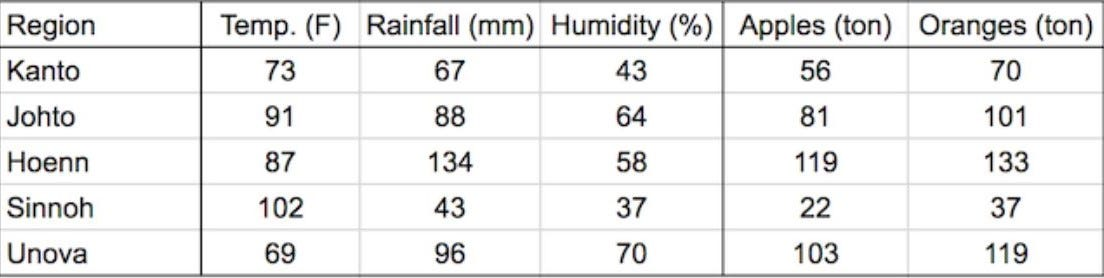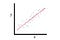# Introduction to Linear Regression

Live as if you were to die tomorrow. Learn as if you were to live forever. - Mahatma Gandhi

Hi !!!!!

Well… this is my first story on medium and I am very excited to share my work on linear regression, an important supervised machine learning algorithm which is used to predict the data.

Hmm… I’m gonna tell your future and I will tell what’s gonna happen to you tomorrow after you wake up! Ha ha just kidding. Wouldn’t it be cool to know what will happen even before the events had happened? I often think about what would happen if we already know the future. Okay I get it. We are into science fiction now and it is not possible to tell the future. But… wait! don’t be sad. What if we could predict near future on small scale events such as stock market, crop production, whether a person is prone to heart disease or not etc. Now that is where machine learning comes into picture.

Machine learning is used to predict the data making use of already existing data. We train our machine learning model on the existing data to make our prediction. Of course the prediction might not be accurate, but it is important to know about the predicted data so that we can analyse the things to make our future results even better.

With all that being said let us see a practical approach of predicting data using linear regression algorithm.

# Linear Regression

Now, let us consider an example showing us the yield of apples and oranges in five different regions along with the regions’ temperature, rainfall, humidity. This is called as training data.Training data

The yield of apples and oranges in a region depends on the temperature, rainfall and humidity of that particular region. So, we can say that temperature, rainfall, humidity are the independent variables and yield of apples and oranges are the dependent variables. In machine learning we have to use this data to predict the yield of apples and oranges in a new region. In this article we will use the linear regression algorithm to predict the yield of apples and oranges in a new region.Simple Linear Regression

This is how a simple linear regression plot would look like. The straight line is called as ‘the best fit line’ which is used to predict the data. It expresses the best relationship between the variables x and y. The equation of a straight line can be expressed as:

y = m*x + c

where x, y are the co-ordinates, ‘m’ is the slope of the line and ‘c’ is the y intercept.

In linear regression the target variable is expressed as the weighted sum of input variables and some constant offset known as bias.

y = B1*x1 + B2*x2 + B3*x3 + ……. Bi*xi + bias

where Bi is the weight, xi is the input and y is the output.

We can write the same kind of equation for our training data which includes inputs which are temperature, rainfall, humidity and outputs that are yield of apples and oranges and their respective weights and biases.

`yield_apple  = w11 * temp + w12 * rainfall + w13 * humidity + b1yield_orange = w21 * temp + w22 * rainfall + w23 * humidity + b2`

‘w’ is the weight and ‘b’ is the bias

The graphical representation of inputs versus output would look as:

Here we excluded the humidity for simplicity of the graph.

The prediction depends on adjusting the values of weights and biases. Using weights and biases we could predict the yield of apples and oranges of a new region using the average temperature, rainfall and humidity of that region.

Now, let us start training our model by importing NumPy and PyTorch libraries.

`import numpy as npimport torch`

Now, we have to separate the training data into input and output matrices

`# Input (temp, rainfall, humidity)inputs = np.array([[73, 67, 43],                    [91, 88, 64],                    [87, 134, 58],                    [102, 43, 37],                    [69, 96, 70]], dtype='float32')inputsarray([[ 73.,  67.,  43.],       [ 91.,  88.,  64.],       [ 87., 134.,  58.],       [102.,  43.,  37.],       [ 69.,  96.,  70.]], dtype=float32)# Targets (apples, oranges)targets = np.array([[56, 70],                     [81, 101],                     [119, 133],                     [22, 37],                     [103, 119]], dtype='float32')targetsarray([[ 56.,  70.],       [ 81., 101.],       [119., 133.],       [ 22.,  37.],       [103., 119.]], dtype=float32)array([[ 56.,  70.],       [ 81., 101.],       [119., 133.],       [ 22.,  37.],       [103., 119.]], dtype=float32)`

Now, we have to convert these NumPy arrays into PyTorch tensors.

`# Convert inputs and targets to tensorsinputs = torch.from_numpy(inputs)targets = torch.from_numpy(targets)print(inputs)print(targets)tensor([[ 73.,  67.,  43.],[ 91.,  88.,  64.], [ 87., 134.,  58.], [102.,  43.,  37.], [ 69.,  96.,  70.]])tensor([[ 56.,  70.], [ 81., 101.], [119., 133.],[ 22.,  37.], [103., 119.]])`

Now, we have to create matrices for weights and biases

`# Weights and biasesw = torch.randn(2, 3, requires_grad=True)b = torch.randn(2, requires_grad=True)print(w)print(b)tensor([[-0.5494, -1.0288,  0.7763], [-0.0694, -0.9043, -0.4502]], requires_grad=True) tensor([0.7394, 0.3422], requires_grad=True)`

torch.randn creates a tensor with given shape where elements are picked randomly from a normal distribution with mean zero and standard deviation as one.

Our model is a function that performs a matrix multiplication of the input matrix and the transpose of weights matrix and adds the bias matrix for each output. This output is the predicted matrix and we have to compare it with the actual output or target matrix.

`inputs @ w.t() + btensor([[ -74.9169,  -84.6709],        [ -90.1092, -114.3642],        [-139.8958, -152.9829],        [ -70.8148,  -62.2804],        [ -81.5957, -122.7720]], grad_fn=<AddBackward0>)`

@ represents matrix multiplication in PyTorch and the .t method returns the transpose of a tensor. Now this matrix is the prediction matrix. Let us implement the above matrix operation with the help of a function.

`def model(x):    return x @ w.t() + b# Generate predictionspreds = model(inputs)print(preds)tensor([[ -74.9169,  -84.6709], [ -90.1092, -114.3642], [-139.8958, -152.9829], [ -70.8148,  -62.2804], [ -81.5957, -122.7720]], grad_fn=<AddBackward0>)`

Here we are passing the input matrix to the function model which returns us the prediction matrix.

Now, let us compare the predicted matrix with actual output or target matrix.

`# Compare with targetsprint(targets)tensor([[ 56.,  70.], [ 81., 101.], [119., 133.], [ 22.,  37.], [103., 119.]])`

The Loss Function

Let us see the difference between our predictions and the actual outputs.

`diff = preds - targetsdifftensor([[  2.0911,   2.3917], [  6.1790,  -2.6057], [-13.1012,   1.7874], [  4.1791,  11.4107], [  4.7419, -10.4028]], grad_fn=<SubBackward0>)`

We have to reduce this difference to predict the data accurately.

Before we improve our model, we need a way to evaluate how well our model is performing.

Calculate the difference between the two matrices (preds and targets). Squaring all the elements of the difference matrix to remove negative values. Calculate the average of the elements in the resulting matrix. The result is a single number, known as the mean squared error (MSE).

Let us try to implement the MSE

`# MSE lossdef mse(t1, t2):    diff = t1 - t2    return torch.sum(diff * diff) / diff.numel()# Compute lossloss = mse(preds, targets)print(loss)tensor(37653.6133, grad_fn=<DivBackward0>)`

Gradient Descent

Gradient descent is an algorithm to find the minimum value of the mean squared error. It involves calculating the gradients and apply them to our model. We can calculate gradients w.r.to weights and biases with the help of PyTorch. Here we calculating gradient or derivative of loss function w.r.t weights and biases.

`# Compute gradientsloss.backward()# Gradients for weightsprint(w)print(w.grad)tensor([[ 0.5502, -1.0457, -0.3085], [ 1.8869,  1.2371,  0.8541]], requires_grad=True)tensor([[-11202.0654, -13900.5625,  -8207.4629],         [ 18944.8359,  19087.8008,  11991.8184]])print(b)print(b.grad)tensor([-1.6014,  1.6320], requires_grad=True) tensor([-137.6575, 221.2482])`

Now our objective is to find a set of weights and biases that minimizes the loss of our model. Some important points to remember is that:

If the gradient element is positive; increase in weight increases the loss and vice-versa.

If the gradient element is negative; increase in weight decreases the loss and vice-versa.

The increase or decrease in loss by a weight element is proportional to gradient of loss w.r.to that element. This is the main observation of gradient descent.

Now, to decrease the loss we can subtract a small quantity proportional to the derivative of the loss w.r.to that element.

`with torch.no_grad():    w -= w.grad * 1e-5    b -= b.grad * 1e-5w, b(tensor([[ 0.6622, -0.9067, -0.2264],         [ 1.6975,  1.0462,  0.7342]], requires_grad=True), tensor([-1.6000,  1.6298], requires_grad=True))`

We are multiplying the gradient with a small amount so that we descend down the hill very slowly and not with giant leaps so that we do not miss the optimum minimal value of the loss. This multiplication factor is called as learning rate.

`# Let's verify that the loss is actually lowerpreds = model(inputs)loss = mse(preds, targets)print(loss)tensor(25429.4922, grad_fn=<DivBackward0>)`

Now, we have to reset the gradients to zero. We do this because PyTorch accumulates the gradients. If you try to perform the gradient descent without resetting it would throw unexpected results.

`w.grad.zero_()b.grad.zero_()print(w.grad)print(b.grad)tensor([[0., 0., 0.], [0., 0., 0.]])tensor([0., 0.])`

So, now the gradients being reset to zero, let us start training our model.

Training the model

Let us see some of the steps involved in training the model:

1. Generating predictions.
2. Calculating the loss.
3. Computing gradients.
4. Performing gradient descent.
5. Reset gradients to zero.

Now, to implement them we have to run epochs or iterations so that our loss becomes minimum with every epoch.

Let us train our model for 100 epochs.

`# Train for 100 epochsfor i in range(300):    preds = model(inputs)    loss = mse(preds, targets)    loss.backward()    with torch.no_grad():        w -= w.grad * 1e-5        b -= b.grad * 1e-5        w.grad.zero_()        b.grad.zero_()# Calculate losspreds = model(inputs)loss = mse(preds, targets)print(loss)tensor(58.6509, grad_fn=<DivBackward0>)`

Now, the loss is much lower compared to the initial epoch.

Let us see our predictions and compare them with actual outputs.

`# Predictionspredstensor([[ 59.5036,  72.5822],        [ 81.9154,  98.2326],        [115.5916, 134.8530],        [ 36.0425,  48.2576],       [ 92.3665, 108.6549]], grad_fn=<AddBackward0>)# Targetstargetstensor([[ 56.,  70.],        [ 81., 101.],        [119., 133.],        [ 22.,  37.],        [103., 119.]])`

As you can see that by training our model many a times the loss became much lower and our predictions became much closer to the actual outputs.

Now let us try to predict the yield of apples and oranges in a new region by providing the averages of temperature, rainfall and humidity of that region as inputs to our model.

`# Providing the necessary inputs to predict yield of apples and orangesmodel(torch.tensor([[75, 63, 44.]]))`

Now, let us have a look at our predictions. Man… I’m so excited!

`tensor([[56.6015, 69.5131]], grad_fn=<AddBackward0>)`

This is the predicted yield of apples and oranges of the new region.

Yeah!!!

We have predicted the yield in a new region by making use of already existing data. Now, I’m going to predict your future and tell what’s going to happen to you tomorrow. Ha ha don’t afraid it’s just a joke. We can run our model for even more epochs to reduce the loss and make our predictions even mor e accurate. So, now I have told you the secret of how we can actually predict data!

But wait… I’m gonna tell you something. In real world the outcome we are trying to predict might depend on so many features or inputs and prediction becomes quite difficult. We need large amounts of data to predict in real world. Hmm… don’t worry that’s what we do in machine learning and by using various algorithms we could predict or classify with more accuracy.

# Conclusion

A curious mind that is trying to make use of everyday to learn new stuff.

## More from Putheti Vamsi Krishna

A curious mind that is trying to make use of everyday to learn new stuff.